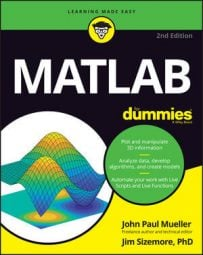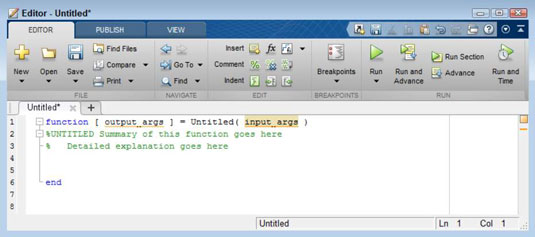##### MATLAB For DummiesMany elegant programming techniques exist in MATLAB, but none are quite so elegant as the recursive function. You create a function that keeps calling itself until a condition is satisfied, and then the function delivers an answer based on the results of all those calls. This process of the function calling itself multiple times is known as recursion, and a function that implements it is a recursive function.

The most common recursion example is calculating factorial (n!), where n is a positive number. (Calculating a factorial means multiplying the number by each number below it in the hierarchy. For example, 4! is equal to 4*3*2*1 or 24.)

Most examples that show how to create a recursive function don’t really demonstrate how the process works. The following steps help you create a recursive function that does demonstrate how the process works.

1. Click the arrow under the New entry on the Home tab of the MATLAB menu and select Function from the list that appears.

You see the Editor window.2. Change output_args to Result.

The function returns a result to each preceding cycle of the call.

3. Change the function name from Untitled to Factorial1.

The primary function name must match the name of the file.

4. Change input_args to Value, Level.

The Value received is always one less than the previous caller received. The Level demonstrates how Value is changing over time.

5. Type the following code into the function between the comment and the end keyword.

```if nargin < 2
Level = 1;
end
if Value > 1
fprintf(‘Value = %d Level = %dn’, Value, Level);
Result = Factorial1(Value - 1, Level + 1) * Value;
disp([‘Result = ‘, num2str(Result)]);
else
fprintf(‘Value = %d Level = %dn’, Value, Level);
Result = 1;
disp([‘Result = ‘, num2str(Result)]);
end```

This example makes use of an optional argument. The first time the function is called, Level won’t have a value, so the application automatically assigns it a value of 1.

The code breaks the multiplication task into pieces. For example, when Value is 4, the code needs to multiply it by 3 * 2 * 1. The 3 * 2 * 1 part of the picture is defined by the call to Factorial1(Value - 1, Level + 1).

During the next pass, Value is now 3. To get the appropriate result, the code must multiply this new value by 2 * 1. So, as long as Value is greater than 1 (where an actual result is possible), the cycle must continue.

A recursive function must always have an ending point — a condition under which it won’t call itself again. In this case, the ending point is the else clause. When Value is finally less than 1, Result is assigned a value of 1 and simply returns, without calling Factorial1() again. At this point, the calling cycle unwinds and each level returns, one at a time, until a final answer is reached.

Notice that this example uses a new function, fprintf(), to display information onscreen. The fprintf() function accepts a formatting specification as its first input. In this case, the specification says to print the string Value =, followed by the information found in Value, then Level =, followed by the information found in Level.

The %d in the format specification tells fprintf() to print an integer value. You use fprintf() as a replacement for disp() when the output formatting starts to become more complex. Notice that disp() requires the use of the num2str() function to convert the numeric value of Result to a string in order to print it.

6. Click Save.

You see the Select File for Save As dialog box. Notice that the File Name field has the correct filename entered for you.7. Click Save.

The function file is saved to disk.

8. Type Factorial1(4) and press Enter in the Command window.

You see the following output:

```Value = 4 Level = 1
Value = 3 Level = 2
Value = 2 Level = 3
Value = 1 Level = 4
Result = 1
Result = 2
Result = 6
Result = 24
ans =
24```

Notice that all the Value and Level outputs come first. The function must keep calling itself until Value reaches 1. When Value does reach 1, you see the first Result output. Of course, Result is also 1. Notice how the recursion unwinds. The next Result is 2 * 1, then 3 * 2 * 1, and finally 4 * 3 * 2 * 1.

Now that you have a better idea of how the recursion works, look at the slimmed-down version.

```function [ Result ] = Factorial2( Value )
%Factorial2 - Calculates the value of n!
% Outputs the factorial value of the input number.
if Value > 1
Result = Factorial2(Value - 1) * Value;
else
Result = 1;
end
end```

The final version is much smaller but doesn’t output any helpful information to tell you how it works. Of course, this version will run a lot faster, too.